• # Python学习系列之运算符及运算符的优先级（六）

一、Python中的运算符包括：

算术运算符
赋值运算符
比较运算符
布尔运算符
位运算符
其中算术运算符包含：标准算术运算符，取余运算符（%）、幂运算符（**）

标准运算符包括：加（+）、减（-）、乘（*）、/、整除（//）

1、Python算术运算符的使用

`#加法运算print(1+1)#减法运算print(4-1)#乘法运算print(3*2)#除法运算print(11/2)#整除运算print(11//2)#取余运算print(11%2)#幂运算print(11**2)    #表示11的2次方`

以上执行结果如下：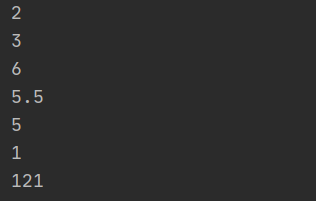解析：当出现除数和被除数为负数是，会有两种情况，一种是同为负数或一负一正，当一负一正时整除，会获取整数值，当同为负数时，

整除运算有负数情况举例：

```print(9//4)     #同为正数，取整
print(-9//-4)   #同为负数，取整
print(9//-4)    #一正一负整除，向下取整，除的结果是-2.25，所以结果是-3
print(-9//4)    #一正一负整除，向下取整，除的结果是-2.25，所以结果是-3
```

执行结果如下：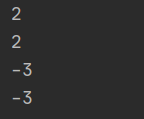取余运算有负数情况举例：

```print(9%-4)     #值为-3，计算公式：余数=被除数-除数*商  9-(-4)*(-3)=9-12=-3
print(-9%4)     #值为3，计算公式：余数=被除数-除数*商  (-9)-(4)*(-3)=(-9)-(-12)=(-9)+12=3
```

运行结果如下图：2、Python赋值运算符

赋值运算符
执行顺序：右到左
支持链式赋值，如a=b=c=0
支持参数赋值，如+=、-=、*=、/=、//=、%=
支持系列解包赋值，a,b,c=10,20,30

举例：

```#赋值运算符
i=3+5
print(i)

#链式赋值
a=b=c=10
print(a,id(a))
print(b,id(b))
print(c,id(c))
```

执行结果如下：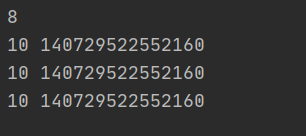解析：1、i=3+5，首先将3+5进行运算得出8，再将8赋值给变量i

2、将a、b、c三个变量都赋值为10，这三个变量值都是10，同时指向同一个内存空间

如果将链式赋值分开写，也会是同样的结果，即都是执行同一个内存空间，该内存空间的值是10

```a=10
b=10
print(a,id(a))
print(b,id(b))
```

执行结果如下：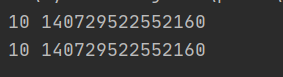2、参数赋值

`#参数赋值`
`a=30a+=20   #a=a+20print(a,type(a))a-=10   #a=a-10print(a,type(a))a*=2    #a=a*2print(a,type(a))a/=2    #a=a/2print(a,type(a))a//=2   #a=a//2print(a,type(a))a%=2    #a=a%2print(a,type(a))`

执行结果如下：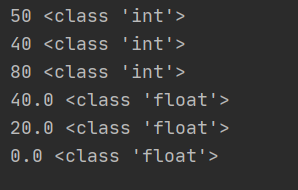3、解包赋值

3.1 常用模式a,b,c=10,20,30,意思是将10赋值给a，将20赋值给b,将30赋值给c

举例：

```a,b,c=10,20,30
print(a,b,c)
```

执行结果如下：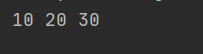即分别将10赋值给a，将20赋值给b,将30赋值给c

3.2 解包赋值要求=号两边的数量保持一致

举例：

```a,b=10,20,30
```

执行结果如下：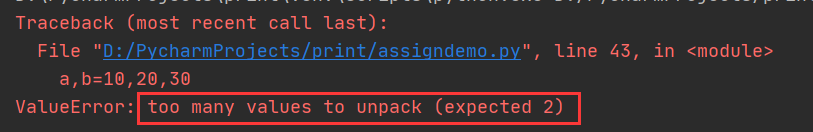由此可以看出，当使用解包赋值时，两边的变量或者值不一致是会报错

3.3 解包赋值可以直接交换两个变量的值，不需要中间变量

举例：

```#解包赋值可以交换两个变量的值
a,b=10,20
print('交换之前的值：',a,b)
a,b=b,a
print('交换之后的值：',a,b)
```

执行结果如下：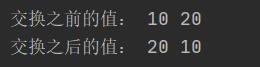解析：直接将变量赋值按位置赋值给对应的变量，无需中间变量

4、Python中的比较运算符

4.1 比较运算符输出的是bool值，即是比对是对（True）还是错(False)

举例：

```#比较运算符
a,b=10,20
print('a>b吗？',a>b)
print('a<b吗？',a<b)
print('a<=b吗？',a<=b)
print('a>=b吗？',a>=b)
print('a=b吗？',a==b)
print('a!=b吗？',a!=b)　　```

执行结果如下：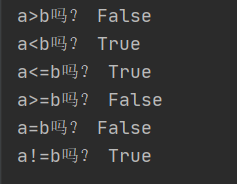4.2 比较运算是比较值还是比较存储标识？

```'''
一个 = 称为赋值运算符，==称为比较运算符
一个变量由三部分组成，标识、类型、值
== 比较的是值还是标识呢？ 比较的是值
比较对象的标识使用 is

'''```
`a=10b=10print(a==b)print(id(a))print(id(b))print(a is b)print(a is not b)`

以上代码执行结果是：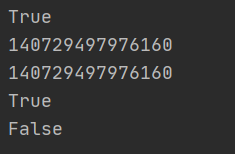解析：1.a和b的值相同，都是10

2.a和b的内存空间是同一个，即内存标识是同一个，因为内存中已经有一个一模一样的值的存储空间，不会再新建一个空间，而是直接将变量的标识符指向已有的内存空间，所以a和b的内存标识是一样的，所以比对结果是True

3.a和b的内存空间是同一个，所以is not比对结果是False

4.3 两个值相同的List列表会是同一个空间吗？

举例：

```#比对list的值和内存空间list1=[11,22,33,44]
list2=[11,22,33,44]
print(list1==list2)
print(list1 is list2)
print(id(list1))
print(id(list2))
```

执行结果如下：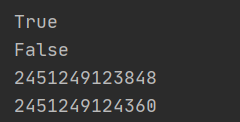解析：1.list1和list2的值是相同的，所以比对结果是True

2.list1和list2的内存空间id是不相同的，所以结果是False

5、bool运算符

bool运算符有：and、or、not、in、not in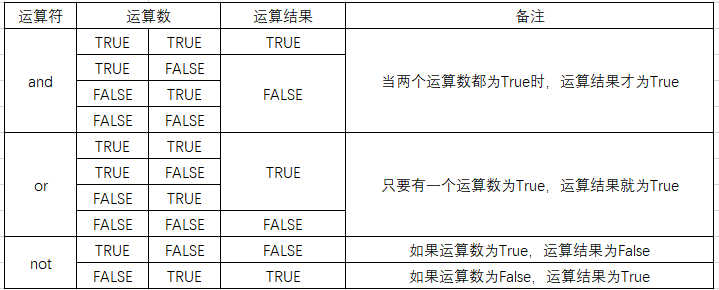5.1、bool运算and运算

举例：

```#bool运算符and运算
a,b=1,2
print(a==1 and b==2)       #True and True --> True
print(a==1 and b<2)        #True and False --> False
print(a!=1 and b==2)       #False and True --> False
print(a!=1 and b!=2)       #False and False --> False
```

执行结果如下图：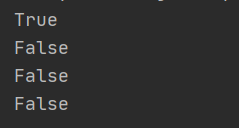解析：and运算只有当两个都是True时，才是True，只要有一个False则结果都是False。and可以理解为并且或者全部。

5.2、bool运算符or运算

举例：

```#bool运算符or运算
a,b=1,2
print(a==1 or b==2)       #True and True --> True
print(a==1 or b<2)        #True and False --> True
print(a!=1 or b==2)       #False and True --> True
print(a!=1 or b!=2)       #False and False --> False
```

执行结果如下：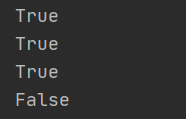解析：or运算中，当其中一个运算为True时结果为True，两个值都为False时才为False。or可以理解为或。

5.3、bool运算符not运算

举例：

```#bool运算符not运算,即取反
a1=True
a2=False
print(not a1)
print(not a2)
```

执行结果如下：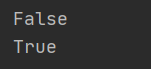解析：a1的值为True，取反的结果就是False，a2的值是False，取反的结果就是True

5.4 bool运算符in、not in运算，即是否在里面

举例：

```#bool运算符in、not in运算,即是否在里面
a='hellworld'
print('h' in a)
print('k' in a)
print('h' not in a)     #False
print('k' not in a)     #True
```

执行结果如下：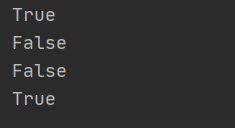解析：1. h在helloworld单词里，则in运算执行结果为True，not in运算结果为False

2. k不在helloworld单词里，则执行结果为False，not in运算结果为True

6、位运算符

位运算符有位与&、位或|、左移、右移。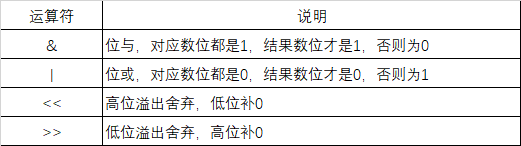6.1 按位与和按位或举例：

```#位运算
print(4&8)      #按位与&，同为1时结果为1，否则为0
print(4|8)      #按位或|，同为0时结果为0，否则为1
```

执行结果为：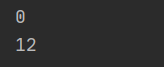解析：1.4的二进制00000100，8的二进制是00001000，按位与的结果是00000000即0，按位或的结果是00001100即12

6.2 左右位移运算：

图解：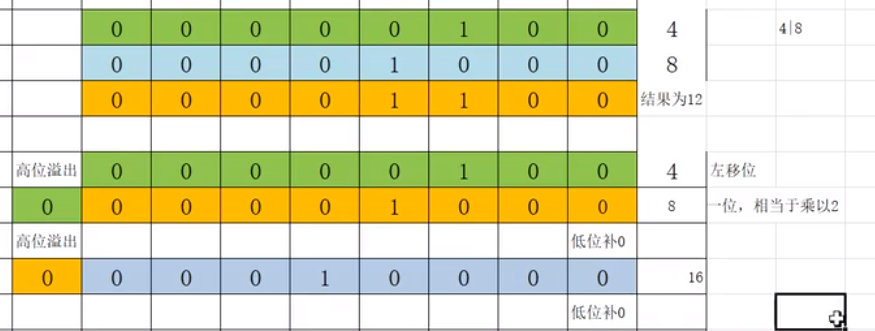解析：4的二进制是0000100，左位移时高位溢出一位，低位少一位需要补零，4左位移的结果是00001000即是8，左位移一位相当于乘以2

8的二进制是00001000，再左位移时高位溢出一位，低位少一位需补零，8左位移的结果是00010000即16，左位移一位相当于乘以2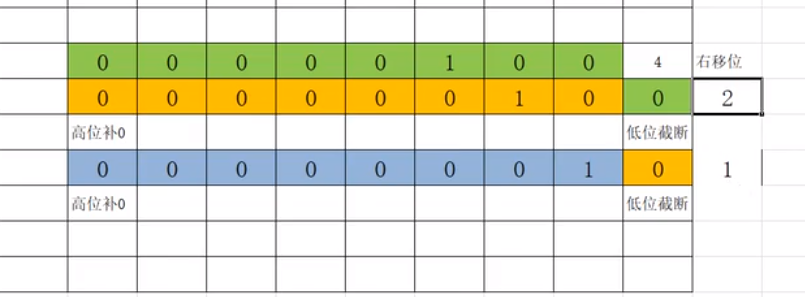解析：4的二进制是0000100，右位移时低位截断一位，高位少一位需要补零，4右位移的结果是00000010即是2，右位移一位相当于除以2

2的二进制是00000010，再右位移时低位截断一位，高位少一位需要补零，2右位移的结果是00000001即1，右位移一位相当于除以2

代码举例：

```#左右位移
print(4<<1)     #4左移一位，相当于乘以2，结果是8
print(4<<2)     #4左移二位，相当于乘以4，结果是16
print(4>>1)     #4右移一位，相当于除以2
print(4>>2)     #4右移二位，相当于除以4
```

执行结果如下：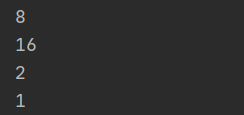7. 运算符的优先级

运算符优先级规则：

1、有括号先计算括号

2、优先级第二高的是算术运算符，算术运算符中优先计算乘除，再计算幂，再计算加减

3、优先级第三高的是位运算符，先计算位移，再计算按位或，最后计算按位与

4、优先级第四高的是比较运算，比较运算的结果是bool值

5、优先级最低的是bool运算，优先and和赋值运算，再执行or运算

• 相关阅读:
RBAC-基于角色的访问控制
django缓存机制
drf JWT认证
drf自动生成接口文档
drf多表断表操作
drf过滤排序分页异常处理
drf认证权限频率
drf路由组件
drf视图组件
drf请求与响应
• 原文地址：https://www.cnblogs.com/wx170119/p/14004476.html